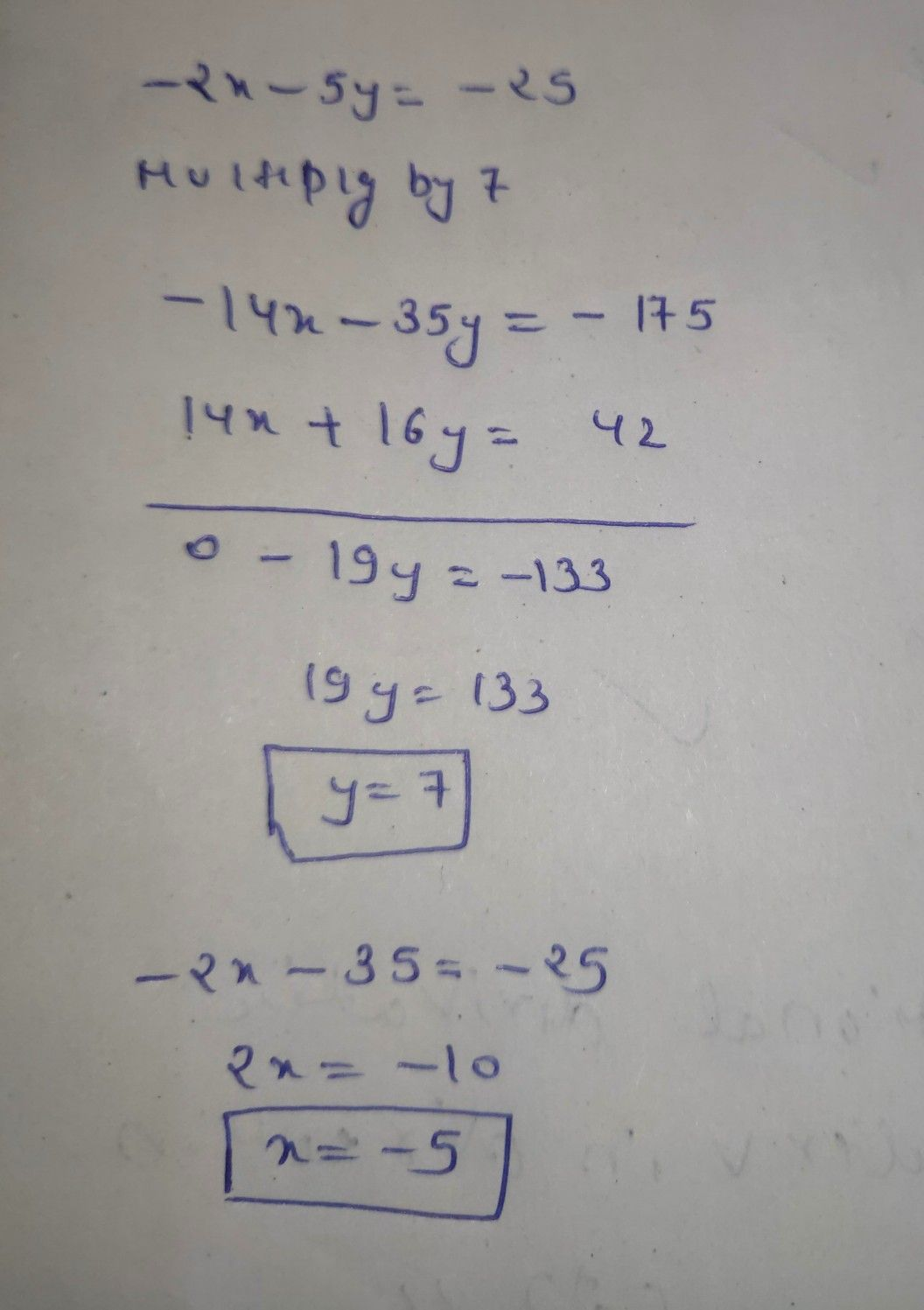Symbol
ProblemIncorrect Your answer is incorrect. • X: Your answer is incorrect. $y=$ Your answer is incorrect. Solve the following system of equatio $-2x-5y=-25$ $7x+8y=21$ $x=\left($ $1=|\right)$ $\bar{5}$